﻿ Reliability Level of Welding Voltage Dependence of Heat Affected Zone (HAZ) Hardness of Selected Metallic Weldments Cooled in Groundnut Oil

Reliability Level of Welding Voltage Dependence of Heat Affected Zone (HAZ) Hardness of Selected Met...

C. I. Nwoye, C. C. Nwogbu, A. O. Agbo, J. U. Odo, S. O. NwakpaOPEN ACCESSPEER-REVIEWED

Reliability Level of Welding Voltage Dependence of Heat Affected Zone (HAZ) Hardness of Selected Metallic Weldments Cooled in Groundnut Oil

C. I. Nwoye1,, C. C. Nwogbu2, A. O. Agbo3, J. U. Odo1, S. O. Nwakpa1

1Department of Metallurgical and Materials Engineering, Nnamdi Azikiwe University, Awka, Nigeria

2Science Technical Vocation Schools Management Board

3Department of Metallurgical and Materials Engineering, Enugu State University of Science & Technology, Enugu, Nigeria

Abstract

This paper showcases the reliability level associating welding voltage dependence of heat affected zone (HAZ) hardness of aluminium, cast iron and mild steel weldments cooled in groundnut oil. These materials were welded using shielded metal arc technique and the HAZ hardness of the various groundnut oil cooled weldments determined. Three models were derived and used as tools for the empirical analysis of the influence of welding voltage on the HAZ hardness of the weldments. The results of the analysis show that the HAZ hardness of weldments is significantly and reliably affected by the operational welding voltage. Results evaluations largely show that on welding aluminium, cast iron and mild steel, and similarly cooling their respective weldments in groundnut oil, the model: ξ=[(ηv/(η+v))((βaβm+βaβc)/βcβm)0.2396], empirically predicts aluminium weldment HAZ hardness as equivalent of HAZ hardness interaction between cast iron and mild steel. Aluminium weldment HAZ hardness was evaluated as a product of a multiplication operation between the general voltage product rule (GVPR) ((βa βm + βa βc)/ βc βm)0.2396 and the ratio; HAZ hardness product of cast iron and mild steel/ HAZ hardness sum of cast iron and mild steel (ην /( η + ν )). Predicted values of the HAZ hardness of cast iron and mild steel were comparatively analyzed and also found very reliably dependent on the GVPR which is a collective function of their respective welding voltage. The validity of the model was rooted on the core model expression; (βa c + βa/βm ) = (ζ /ν + ζ / η)4.1737 where both sides of the expression were correspondingly equal. Computational analysis of generated results shows that aluminium, cast iron & mild steel weldment HAZ hardness per unit welding voltage as evaluated from experiment and derived model were 1.4714, 4.1818 & 2.3318 (VHN)V-1 and 1.4714, 4.1821 & 2.3319 (VHN)V-1 respectively. Deviational analysis indicates that the maximum deviation of model-predicted HAZ hardness from the experimental results is less than 0.006%. This translates into over 99.99% operational confidence and reliability level for the derived models and over 0.9999 reliability coefficient for the welding voltage dependence of HAZ hardness.

At a glance: Figures

1
Prev Next

• Nwoye, C. I., et al. "Reliability Level of Welding Voltage Dependence of Heat Affected Zone (HAZ) Hardness of Selected Metallic Weldments Cooled in Groundnut Oil." Materials Science and Metallurgy Engineering 1.2 (2013): 37-41.
• Nwoye, C. I. , Nwogbu, C. C. , Agbo, A. O. , Odo, J. U. , & Nwakpa, S. O. (2013). Reliability Level of Welding Voltage Dependence of Heat Affected Zone (HAZ) Hardness of Selected Metallic Weldments Cooled in Groundnut Oil. Materials Science and Metallurgy Engineering, 1(2), 37-41.
• Nwoye, C. I., C. C. Nwogbu, A. O. Agbo, J. U. Odo, and S. O. Nwakpa. "Reliability Level of Welding Voltage Dependence of Heat Affected Zone (HAZ) Hardness of Selected Metallic Weldments Cooled in Groundnut Oil." Materials Science and Metallurgy Engineering 1, no. 2 (2013): 37-41.

 Import into BibTeX Import into EndNote Import into RefMan Import into RefWorks

1. Introduction

It has been widely accepted that applicability of a particular metal and alloy in the construction and fabrication industries is largely dependent on their respective weldability. Furthermore, the strength and hardness of the heat affected zone (HAZ) (in metals and alloys weldments) are significantly important weldability parameters that go a long way determining where these materials can be exactly useful when carrying out design for fabrication. The extent of formation of cold cracks in the coarse-grained region (adjacent to the fusion zone) of the HAZ depends on the hardness of the region. These cracks are formed as the weld cools and resides in the HAZ even before the section is put into service. Studies [1, 2] have reported that even though cold cracks formation must be avoided, HAZ can be limited by specification.

The hardening allowed in HAZ depends on several factors: the hydrogen level (determined by the welding process used), joint preparation and work piece thickness, and most importantly the metal composition, specifically the equivalent carbon content.

Apart from formation of cold cracks, the corrosion sensitivity of the HAZ is also dependent on its hardness.

The growing need in design and fabrication practice to develop reliable methods of calculating HAZ hardness level has lead to the derivation of some semi-empirical models [3-9]. These models were based on regression analysis and there are a lot of uncertainties regarding their respective applicability.

Predictability of HAZ hardness due to welding process is very complex in the sense that the weldment presents a three-dimensional geometry with temperature-dependent elastic-plastic, stress-strain behaviour. It is strongly believed that a three-dimensional computational analytical method such as Finite Element Analysis for the welding procedure would be too costly for a single analysis and also difficult for use over a wide parameter range.

Recently, series of empirical models [10, 11, 12, 13] were developed. These models successfully predicted the HAZ hardness of aluminium weldment (cooled in a particular medium) based on the HAZ hardness of mild steel and cast iron similar cooled. Generally, the HAZ hardness of any of the metal weldment was predicted based the HAZ hardness of the two other similarly cooled weldments.

Assessment and computational analysis of the hardness of the heat affected zone (HAZ) in aluminum weldment cooled in water has been achieved using a derived . The general model;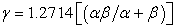(1)

establishes the predictability of the HAZ hardness of aluminum weldment cooled in water as a function of the HAZ hardness of both mild steel and cast iron welded and cooled under the same conditions. The maximum deviations of the model-predicted HAZ hardness values γ, α and β from the corresponding experimental values γexp, αexp and β exp were less than 0.02% respectively.

An intensive research  has been carried out to understand how HAZ hardness of weldment is affected on cooling the weldments in groundnut oil. Models were derived  (using the results of the study) for the evaluation of the HAZ hardness of cast iron weldment cooled in groundnut oil in relation to the respective and combined values of HAZ hardness of aluminum and mild steel welded and cooled under the same conditions. The linear models; α = 2.2330γ, α = 1.7934β and β = 1.2451γ, were found to predict the HAZ hardness of cast iron weldment cooled in groundnut oil as a function of the HAZ hardness of aluminum or mild steel welded and cooled under the same conditions. It was also discovered that the general model;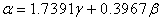(2)

was able to empirically predict the HAZ hardness of cast iron weldment cooled in groundnut oil as eqivalent of the HAZ hardness interaction between aluminum and mild steel welded, since they were all cooled in the same medium under the same conditions. The respective deviations of the model-predicted HAZ hardness values γ, β and α from the corresponding experimental values γexp, βexp, and αexp, was less 0.8% indicating the reliability and validity of the model.

A model  was derived for the predictive analysis of hardness of the heat affected zone in aluminum weldment cooled in groundnut oil. The general model;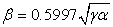(3)

shows that HAZ hardness in aluminium weldment was dependent on the hardness of the heat affected zone (HAZ) in mild steel and cast iron weldments cooled in same media. Re-arrangement of the subject of the model evaluated the HAZ hardness of mild steel α, or cast iron γ respectively as in the case of aluminum.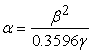(4)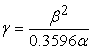(5)

The respective deviations of the model-predicted HAZ hardness values β, γ and α from the corresponding experimental values was less 0.02%.

The purpose of this paper is four-fold: first to describe welding experiments for aluminium, cast iron and mild steel and the resulting HAZ hardness data corresponding to specific welding voltages used; second, to present a simple computational method designed for predicting HAZ hardness of aluminium, cast iron and mild steel weldments cooled in groundnut oil; third to present comparisons between HAZ hardness predicted by the computational model and experimental data; fourth to evaluate the confidence and reliability level for the derived computational model, and also establish the reliability coefficient for the welding voltage dependence of HAZ hardness. These comparisons and evaluations help in the overall assessment of the ability of the computational model to predict the HAZ hardness.

2. Materials and Methods

Mild steel, aluminum and cast iron were cut and welded using the shielded metal arc welding technique and the hardness of the HAZ cooled in groundnut oil (maintained at room temperature) tested. Ten other samples from each of the three materials were also welded, cooled in groundnut oil and their respective HAZ hardness tested. The average HAZ hardness for the weldments of each of the three materials investigated are as presented in Table 2. Table 1 shows the welding currents and voltages used.

3. Model Formulation

Experimental data obtained from the highlighted research work were used for this work. Computational analysis of these data shown in Table 1, gave rise to Table 2 which indicate that;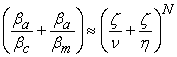(6)

Introducing the value of N into equation (6)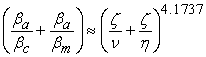(7)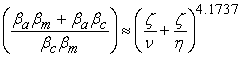(8)

Dividing the indices of both sides of equation (8) by 4.1737;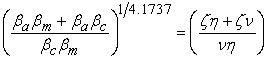(9)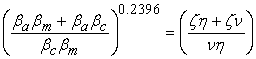(10)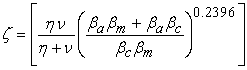(11)

Evaluating the value of η from the derived model in equation (11) gives;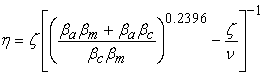(12)

Similarly, from equation (12), ν is evaluated as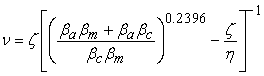(13)

Where

(ζ) = HAZ hardness of aluninium weldment cooled in groundnut oil (VHN)

(ν) = HAZ hardness of cast iron weldment cooled in groundnut oil (VHN)

(η) = HAZ hardness of mild steel weldment cooled in groundnut oil (VHN)

a) = Welding voltage for aluminium (A)

c) = Welding voltage for cast iron (A)

m) = Welding voltage for mild steel (A)

N = 4.1737; equalizing constant

(determined using C-NIKBRAN 

The derived models are equations (11), (12) and (13)

4. Boundary and Initial Conditions

The welding process was carried out under atmospheric condition. After welding, weldments were also maintained at atmospheric condition. In put welding current and voltage range are 120-180A and 220-280V respectively. SiO2-coated electrodes were used to avoid oxidation of weld spots. Welded samples were cooled in groundnut oil which was maintained at 250C. No pressure was applied to the HAZ during or after the welding process. No force due to compression or tension was applied in any way to the HAZ during or after the welding process. The sides and shapes of the samples are symmetries.

5. Results and Discussions

Table 2 shows the variation of materials with the input welding current type (C/Type), welding current (W/C) and voltage (W/V). The result of hardness of the HAZ obtained from aluminium, cast iron and mild steel weldments similarly cooled in groundnut oil (as presented in Table 2) shows that HAZ hardness is greatest in cast iron followed by mild steel, while that of aluminium is lowest.

Table 1. Variation of materials with welding currents and voltages

The derived models are equations (11), (12) and (13). Computational analysis of Table 2 gave rise to Table 3. The precision and validity of the model is stemmed on this table (Table 3).

Table 3. Variation of (βa/βc+βa/βm) with (ξ/v+ξ/η)4.1737

It could be seen from equation (11) that on welding aluminium, cast iron and mild steel, and then cooling their respective weldments similarly in groundnut oil, the HAZ hardness in aluminium weldment is evaluated as equivalent of HAZ hardness interaction between cast iron and mild steel. Aluminium weldment HAZ hardness was evaluated as a product of a multiplication operation between the general current product rule (GCPR) ((βa βm + βa βc)/ βc βm)0.2396 and the ratio; HAZ hardness product of cast iron and mild steel/ HAZ hardness sum of cast iron and mild steel (ην /(η +ν )). Other derived models (equations (12) and (13)) also show the weldment HAZ hardness of each of cast iron and mild steel as equivalent of HAZ hardness interaction between the other two materials HAZ hardness. The predicted HAZ hardness values are all significantly dependent on the GVPR which is a collective function of their respective welding voltages. This implies that ((βa βm + βa βc)/ βc βm)0.2396 is the general voltage product rule and acts as multiplying factor to ην/(η + ν). The highlighted analysis therefore shows that the HAZ hardness of weldments is significantly affected by the input welding voltage.

6. Model Validation

The validity of the derived model was tested by comparing the weldment HAZ hardness of the three materials as evaluated from experiment and derived model. This was done using computational and deviational analysis. The validity of the model was found to be rooted in equation (6) (core of the model) where both sides of the equation are correspondingly equal. Table 3 also agrees with equation (6) following the values of (βa c + βa/βm) and (ζ /ν + ζ / η)4.1737 comparatively evaluated from the experimental results in Table 1.

7. Computational Analysis

A comparative computational analysis of the experimental and model-predicted weldment HAZ hardness per unit welding current were carried out for the three materials to ascertain the degree of validity of the derived model. These were evaluated from calculations involving experimental results, and derived model.

7.1. Aluminium HAZ Hardness per Unit Welding Voltage

Aluminium HAZ hardness per unit welding voltage HAV ; was calculated from the equation;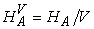(14)

Dividing the HAZ hardness of aluminium weldment; 412 VHN (as shown in Table 2) with the input welding voltage V; 280 V gives the HAZ hardness per unit welding voltage as 1.4714 (VHN) V-1. This is the experimentally obtained aluminium weldment HAZ hardness per unit welding voltage.

Figure 1. Comparison of aluminium HAZ hardness of weldments as obtained from experiment and derived model

Also, dividing the model-predicted HAZ hardness of aluminium weldment; 411.9852 VHN with the input welding voltage; 280 V, the model-predicted aluminium HAZ hardness per unit welding voltage is given as 1.4714 (VHN) V-1.

7.2. Cast Iron HAZ Hardness per Unit Welding Voltage

Cast iron HAZ hardness per unit welding voltage HCV; was calculated from the equation;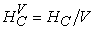(15)

On dividing the HAZ hardness of cast iron weldment; 920 VHN (as shown in Table 2) with the input welding voltage; 220V gives the HAZ hardness per unit welding voltage as 4.1818 (VHN) V-1. This is the experimentally obtained cast iron weldment HAZ hardness per unit welding voltage

Furthermore, dividing the model-predicted HAZ hardness of cast iron weldment; 920.0536 VHN with the input welding voltage; 220V gives 4.1821 (VHN) V-1 as the model-predicted cast iron HAZ hardness per unit welding voltage.

7.3. Mild Steel HAZ Hardness per Unit Welding Voltage

Mild steel HAZ hardness per unit welding voltage HMV; was calculated from the equation;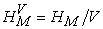(16)

Dividing the HAZ hardness of mild steel weldment; 513 VHN (as shown in Table 2) with the input welding voltage; 220 V gives 2.3318 (VHN) V-1 as the HAZ hardness per unit welding voltage as obtained from experiment.

Figure 3. Comparison of mild steel HAZ hardness of weldments as obtained from experiment and derived model

Similarly, dividing the model-predicted HAZ hardness of mild steel weldment; 513.0121 VHN with the input welding voltage; 220 V gives 2.3319 (VHN) V-1 as the model-predicted mild steel HAZ hardness per unit welding voltage.

An analysis of Figure 1, Figure 2, and Figure 3 shows proximate agreement between HAZ hardness as evaluated from experiment and derived model. A comparison of these three corresponding sets of HAZ hardness values per unit welding current shows proximate agreement and invariably a high degree of validity for the derived model.

8. Deviational Analysis

Comparative analysis of HAZ Hardness from the experiment and derived model revealed very insignificant deviations on the part of the model-predicted values relative to values obtained from the experiment. This is attributed to the fact that the experimental process conditions which influenced the research results were not considered during the model formulation. This necessitated the introduction of correction factor, to bring the model-predicted HAZ hardness results to those of the corresponding experimental values.

Deviation (De) of model-predicted HAZ hardness from that of the experiment is given by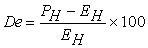(17)

Correction factor (Cr) is the negative of the deviation i.e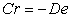(18)

Therefore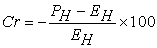(19)

Where

De = Deviation (%)

PH = Model-predicted HAZ hardness (VHN)

EH = HAZ hardness from experiment (VHN)

Cr = Correction factor (%)

Introduction of the corresponding values of Cr from equation (19) into the model gives exactly the corresponding experimental HAZ hardness.

Table 4. Variations of model predicted HAZ hardness with deviations and correction factors

Table 4 indicates clearly that the maximum deviation of model-predicted HAZ hardness (from experimental values) is less than 0.006 %. This is insignificant and very much within the acceptable range of deviation from experimental results. The evaluated maximum deviation translates to over 99.99% operational confidence and reliability level for the derived models and over 0.9999 reliability coefficient for the welding voltage dependence of HAZ hardness.

It is important to state that the deviation of model predicted results from that of the experiment is just the magnitude of the value. The associated sign preceding the value signifies that the deviation is a deficit (negative sign) or surplus (positive sign).

9. Conclusions

Following empirical analysis of the influence of welding voltage on the HAZ hardness of aluminium, cast iron and mild steel weldment, the HAZ hardness were significantly and reliably affected by the operational welding voltage. Results evaluation indicate that on welding aluminium, cast iron as well as mild steel, and then similarly cooling their respective weldments in groundnut oil, the model empirically predicted aluminium weldment HAZ hardness as product of a multiplication operation between the general voltage product rule (GVPR) ((βa βm + βa βc)/ βc βm)0.2396 and the ratio; HAZ hardness product of cast iron and mild steel/ HAZ hardness sum of cast iron and mild steel (ην/( η + ν )). Predicted values of the HAZ hardness of cast iron and mild steel were comparatively analyzed and also found very reliably dependent on the GVPR which is a collective function of their respective welding voltage. The validity of the model was rooted on the core model expression; (βa c + βa/βm) = (ζ /ν + ζ / η)4.1737 where both sides of the expression were correspondingly equal. Computational analysis of generated results shows that aluminium, cast iron & mild steel weldment HAZ hardness per unit welding voltage as evaluated from experiment and derived model were 1.4714, 4.1818 & 2.3318 (VHN)V-1 and 1.4714, 4.1821 & 2.3319 (VHN)V-1 respectively. Deviational analysis indicates that the maximum deviation of model-predicted HAZ hardness from the experimental results is less than 0.006%.This translates into over 99.99% operational confidence and reliability level for the derived models and over 0.9999 reliability coefficient for the welding voltage dependence of HAZ hardness.

References

  Stout, R.D. “Hardness as an index of the Weldability and Service Performance of Steel Weldments”, Welding Research Council (WRC) Bulletin 189, 345 East 47th St, New York, NY 10017.In article  Stout, R.D, Torr, S. S and Doan, G.E (1945) Weld. J. 24:6255.In article  Coe, F.R (1973) “Welding Steels without Hydrogen Cracking” (The Welding Institute, Abingdon, UK,).In article  Beckert, M and Holz, R (1973) Schweiss Technik 23:344.In article  Seyffarth, P. (1979) ibid. 27:58165.In article  Bastien, P., Frollet, J. Maynier, PH. (1970) Metal Construct. Brit. Weld. J. 2:9.In article  Ararat, Y., Nishiguchi, K., Ohji, T and Koshai, N (1979). Trans. Jpn Weld. Res. Inst. 8:43.In article  Terasaki, T., (1981). J. Jpn Weld. Res. Inst. 16:145.In article  Yurioka, N., Ohsita S., and Tamehiro, H. (1981). “Study on Carbon Equivalents to Assess Cold Cracking Tendency and Hardness in Steel Welding”, Proceedings of The Specialist Symposium on Pipeline Welding in the 80s, Melbourne.In article  Nwoye, C. I.., Anyakwo, C. N., E. Obidiegwu., and N. E. Nwankwo (2011). Model for Assessment and Computational Analysis of Hardness of the Heat Affected Zone in Water Cooled Aluminium Weldment Journal of Mineral and Materials Characterization and Engineering. 10(8): 707-715.In article  Nwoye, C. I., (2008). Comparative Studies of the Cooling Ability of Hydrocarbon Based Media and their Effects on the Hardness of the Heat Affected Zone (HAZ) in Weldments. Journal of Metallurgical and Materials Engineering, 3(1):7-13.In article  Nwoye, C. I.., and Mbuka, I. E. (2010). Models for Predicting HAZ Hardness in cast iron Weldment Cooled in Groundnut Oil in Relation to HAZ Hardness of Aluminum and Mild Steel Weldments Cooled in Same Media. Materials Research Innovation. 14(4):312-315.In article CrossRef  Nwoye, C. I., Odumodu, U., Nwoye, C. C., Obasi, G. C., and Onyemaobi, O. O. (2009).Model for Predictive Analysis of Hardness of the Heat Affected Zone in Aluminum Weldment Cooled in Groundnut Oil Relative to HAZ Hardness of Mild Steel and Cast Iron Weldments Cooled in Same Media. New York Science Journal. 2(6):93-98.In article  Nwoye, C. I.. (2008). C-NIKBRAN; Data Analytical Memory (Software).In article PubMed